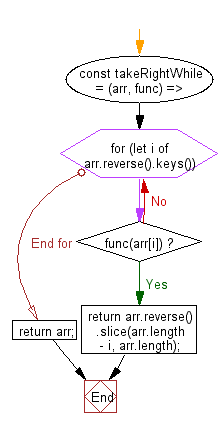# JavaScript: Get removed elements from the end of a given array until the passed function returns true

## JavaScript fundamental (ES6 Syntax): Exercise-129 with Solution

Write a JavaScript program to get removed elements from the end of a given array until the passed function returns true.

• Create a reversed copy of the array, using the spread operator (...) and Array.prototype.reverse().
• Loop through the reversed copy, using a for...of loop over Array.prototype.entries() until the returned value from the function is falsy.
• Return the removed elements, using Array.prototype.slice().
• The callback function, fn, accepts a single argument which is the value of the element.

Sample Solution:

JavaScript Code:

``````//#Source https://bit.ly/2neWfJ2
const takeRightWhile = (arr, func) => {
for (let i of arr.reverse().keys())
if (func(arr[i])) return arr.reverse().slice(arr.length - i, arr.length);
return arr;
};

console.log(takeRightWhile([1, 2, 3, 4], n => n < 3));
```
```

Sample Output:

```[3,4]
```

Flowchart:Live Demo:

See the Pen javascript-basic-exercise-129-1 by w3resource (@w3resource) on CodePen.

Improve this sample solution and post your code through Disqus

What is the difficulty level of this exercise?

Test your Programming skills with w3resource's quiz.

﻿

## JavaScript: Tips of the Day

Chunks an array into n smaller arrays

Example:

```const tips_chunkIntoN = (arr, n) => {
const size = Math.ceil(arr.length / n);
return Array.from({ length: n }, (v, i) =>
arr.slice(i * size, i * size + size)
);
}
console.log(tips_chunkIntoN([1, 2, 3, 4, 5, 6, 7,8], 4));
```

Output:

```[[1,2],[3,4],[5,6],[7,8]]
```